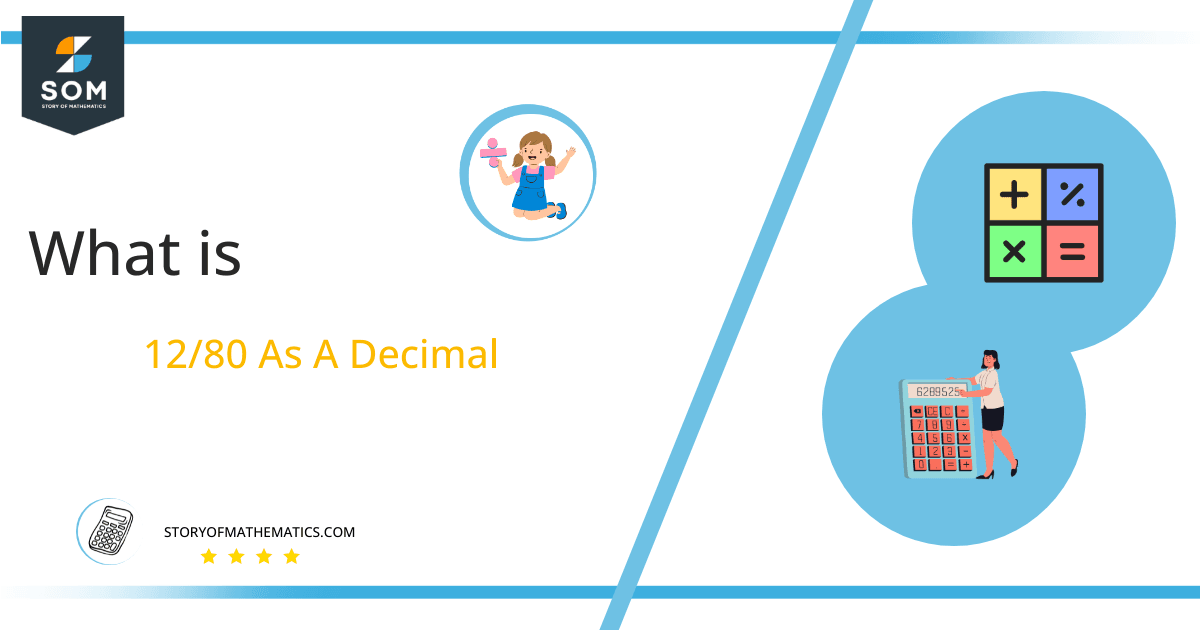# What Is 12/80 as a Decimal + Solution With Free Steps

The fraction 12/80 as a decimal is equal to 0.15.

When you divide two numbers from one another, it gives the result in either fractional value, f/g, or a decimal number, x.yzabc. The numbers after the decimal point are known as the decimals. They can be calculated manually using the long division method.

Here, we are more interested in the division types that result in a Decimal value, as this can be expressed as a Fraction. We see fractions as a way of showing two numbers having the operation of Division between them that result in a value that lies between two Integers.Now, we introduce the method used to solve said fraction to decimal conversion, called Long Division, which we will discuss in detail moving forward. So, let’s go through the Solution of fraction 12/80.

## Solution

First, we convert the fraction components, i.e., the numerator and the denominator, and transform them into the division constituents, i.e., the Dividend and the Divisor, respectively.

This can be done as follows:

Dividend = 12

Divisor = 80

Now, we introduce the most important quantity in our division process: the Quotient. The value represents the Solution to our division and can be expressed as having the following relationship with the Division constituents:

Quotient = Dividend $\div$ Divisor = 12 $\div$ 80

This is when we go through the Long Division solution to our problem. Given is the Long division process in Figure 1:Figure 1

## 12/80 Long Division Method

We start solving a problem using the Long Division Method by first taking apart the division’s components and comparing them. As we have 12 and 80, we can see how 12 is Smaller than 80, and to solve this division, we require that 12 be Bigger than 80.

This is done by multiplying the dividend by 10 and checking whether it is bigger than the divisor or not. If so, we calculate the Multiple of the divisor closest to the dividend and subtract it from the Dividend. This produces the Remainder, which we then use as the dividend later.

Now, we begin solving for our dividend 12, which after getting multiplied by 10 becomes 120.

We take this 120 and divide it by 80; this can be done as follows:

120 $\div$ 80 $\approx$ 1

Where:

80 x 1 = 80

This will lead to the generation of a Remainder equal to 120 – 80 = 40. Now this means we have to repeat the process by Converting the 40 into 400 and solving for that:

400 $\div$ 80 $\approx$ 5

Where:

80 x 5 = 400

Finally, we have a Quotient generated after combining the three pieces of it as 0.15, with a Remainder equal to 0.Images/mathematical drawings are created with GeoGebra.